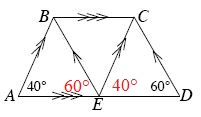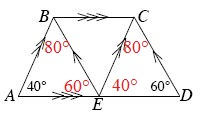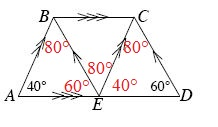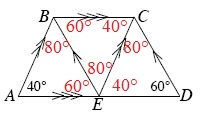### Home > GC > Chapter 5 > Lesson 5.3.5 > Problem5-120

5-120.
1.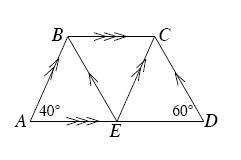trapezoid ABCD at right. Homework Help ✎

1. Find the measures of all the angles in the diagram.

2. What is the sum of the angles that make up the trapezoid ABCD? That is, what is m∡A+ m∡ABC + m∡BCD+ m∡D?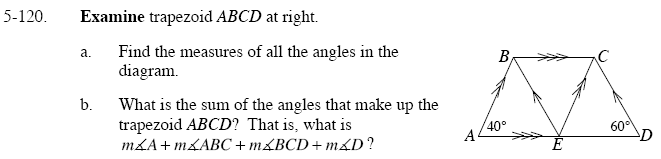The corresponding angles are equal.

Since line AB || line EC, then corresponding angles ∠BAE = ∠CED.
So ∠CED = 40°, mark it on your diagram.
While line CD || line EB, then corresponding angles ∠CDE = ∠BEA.
So ∠BEA = 60°, mark it on your diagram

Use the Triangle Angle Sum Theorem.

Now you know two angles in ΔABE and ΔCED, knowing that one angle is 40° and 60° Calculate the third angle using the equation 40° + 60° + missing angle = 180°: So the missing angle is 80°. Mark it in your diagram.

The angles at E form a straight angle, which is 180º.

Since ∠BEA = 60° and ∠CED = 40° then 40° + 60° + ∠CEB = 180°.
Solve for ∠CEB, mark it on your diagram. ∠CEB = 80°.

The alternate interior angles are equal.

Since ∠BEA = 60° and ∠CED = 40°, then ∠EBC = 60° and ∠ECB = 40° because alternate angles are equal. Mark the angles on your diagram.

40° + (80° + 60°) + (40° + 80°) + 60° = 360°
Note: Do not add in the angles at E.